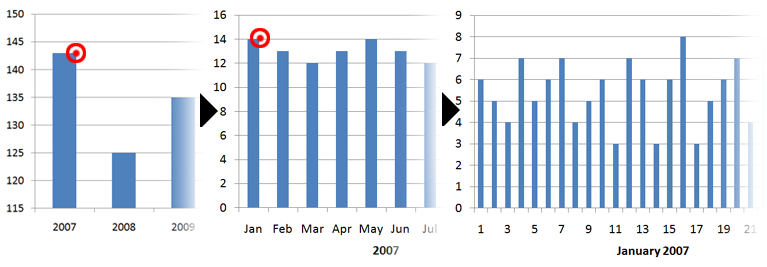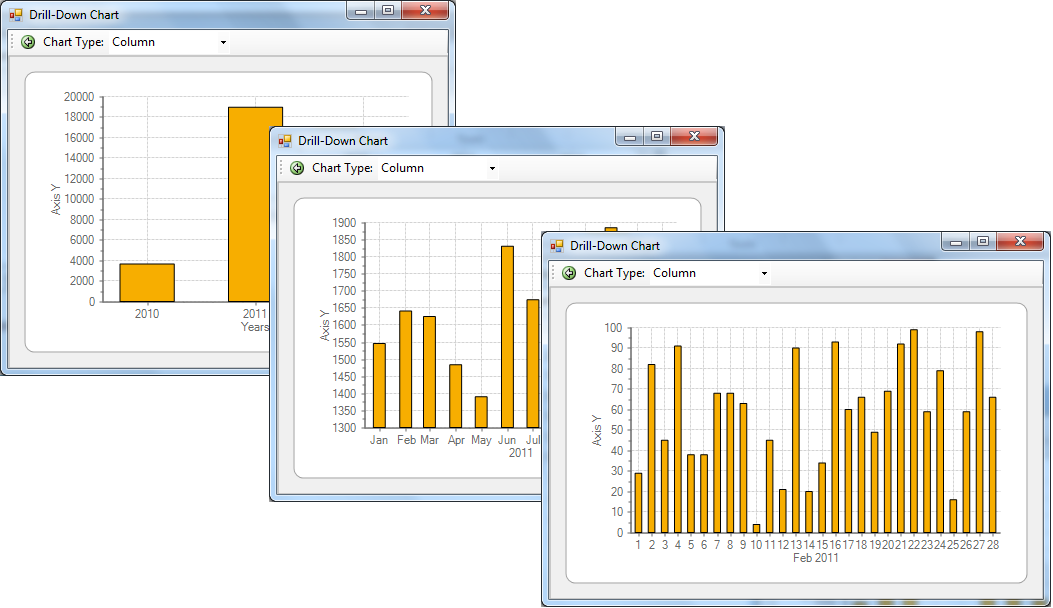﻿ Drill-down Charts using LINQ# Drill-down Charts using LINQ

In this blog sample we'll perform dynamic grouping and summing of data to create drill-down charts using LINQ.

Chart controls are designed to display data in a graphical format. It's not typical for charts to provide advanced data features such as sorting, filtering and grouping. LINQ extends the VB and C# native language syntax for queries allowing us to manipulate our data very easily in code, for things such as filtering and grouping, etc. This makes LINQ the perfect solution for providing drill-down behavior to a chart control.

## What are drill-down charts?

A drill-down chart is one that displays grouped data, and allows users to drill-down into the data for each group. For example, a common drill-down scenario for charts is when we display time on the x-axis and group the data by year, month or day. The data values in each group are totalled up together so we can see the totals per year, month, day, etc. We could perform sums, counts, averages or any aggregation we want. Most likely we will sum the data.In the above picture, the user would click on the 2007 bar and the chart will change to display the data for the year 2007. Then the user can click on a month to drill-down into the days of that month. The UI should provide the user with a way to go back (drill-up?) so he or she can drill-down into a different year or month.

## Using LINQ to group and sum our data

LINQ is the perfect tool to dynamically transform data at runtime, which makes it the perfect solution to add chart grouping functionality. We will build dynamic queries based upon which plot element the user clicks while keeping our original data source intact (raw and unorganized). You can use LINQ to extend objects such as arrays, dictionaries, tables and lists, so ideally your original data source should be of one of these types. For example, here we have a simple list of PlotPoint objects. Each PlotPoint has a Value and a Date.

``````public class PlotPoint
{
public DateTime Date { get; set; }
public double Value { get; set; }
}

List data = new List(); //our original data source
``````

We will fill this collection with some random 500 values.

``````Random rnd = new Random();
for (int i = 0; i < 500; i  )
{
}
``````

Our raw data is ready to go. Next, we will use LINQ extensions on this list to group and sum our data based upon certain filter parameters. In this case, we want to filter our data by year, month or both. If we filter by year then we will be grouping the data by month. If we filter by year and month, then we will group the data by day. If we apply no filter then we can simply group by year. Basically, there are 3 possible conditions.

The GroupData method takes a year and month parameter as integers. Since months are 1-based (January = 1), we can use 0 as a null parameter.

``````private void GroupData(int year, int month)
{
List groupedData = new List();
if (year > 0 && month > 0)
{
//filter on year and month, group by day
groupedData = (from p in data.Where(x => x.Date.Year == year && x.Date.Month == month)
group p by p.Date.Day into d
select new PlotPoint
{
Date = new DateTime(year, month, d.Key),
Value = d.Sum(x => x.Value)
}).ToList();
}
}
``````

That's essentially one line of code in the If statement, and you can see the LINQ syntax for filtering and grouping our collection into a new collection of the same type, named groupedData. We use 'Where' to apply the filter conditions, 'Group by' used with 'Select' gives us a new grouped collection. The power of LINQ also allows us to apply aggregates right in this single line of code! In our Select we are telling the query to sum the values of our PlotPoints for each group. Finally, we call ToList().

We can follow the same LINQ syntax again for our other conditions by just making a few adjustments. Here is the full GroupData method for all 3 conditions.

``````private void GroupData(int year, int month)
{
List groupedData = new List();
if (year == 0 && month == 0)
{
//group and sum all data by year
groupedData = (from p in data
group p by p.Date.Year into d
select new PlotPoint
{
Date = new DateTime(d.Key, 1, 1),
Value = d.Sum(x => x.Value)
}).ToList();
}
else if (year > 0 && month == 0)
{
//group and sum data by month filtered by year
groupedData = (from p in data.Where(x => x.Date.Year == year)
group p by p.Date.Month into d
select new PlotPoint
{
Date = new DateTime(year, d.Key, 1),
Value = d.Sum(x => x.Value)
}).ToList();
}
else if (year > 0 && month > 0)
{
//filter on year and month, group by day
groupedData = (from p in data.Where(x => x.Date.Year == year && x.Date.Month == month)
group p by p.Date.Day into d
select new PlotPoint
{
Date = new DateTime(year, month, d.Key),
Value = d.Sum(x => x.Value)
}).ToList();
}
//TODO: bind chart to groupedData
}
``````

Next, we need to hook this up to our chart!

## Using C1Chart

The key charting requirement for this sample is being able to determine which plot value gets clicked on. With C1Chart this is easy. Then, based upon this value, we will run our LINQ code from above and simply reload the chart. We also need to format the X-Axis to display the new range of values for each view. Finally, we can add history collection and a back button so the user can traverse back like a web browser. To sum up these tasks:

1. Determine plot point user clicks
2. Run LINQ to group/sum data
3. Reload chart with new data
4. Update x-axis labels
5. Store parameters for history

### Determine Plot Point Clicked/Group Data

C1Chart provides several methods we can use in conjuntion with the mouse click event to determine the chart value under the mouse. These methods (CoordToDataIndex, DataIndexToCoord, etc) can return useful information such as the index of the point, series, and distance from the mouse.

``````private void c1Chart1_MouseClick(object sender, MouseEventArgs e)
{
int si = 0; //series index
int pi = 0; //point index
int d = 0; //distance
int year = 0, month = 0;

//Get point at mouse location
if (c1Chart1.ChartGroups.Group0.CoordToDataIndex(e.X, e.Y, CoordinateFocusEnum.XandYCoord, ref si, ref pi, ref d))
{
if (stack.Count == 0)
{
year = 0;
month = 0;
}
else if (stack.Count == 1)
{
year = ((DateTime)c1Chart1.ChartGroups.ChartData[si].X[pi]).Year;
month = 0;
}
else if (stack.Count == 2)
{
year = ((DateTime)c1Chart1.ChartGroups.ChartData[si].X[pi]).Year;
month = ((DateTime)c1Chart1.ChartGroups.ChartData[si].X[pi]).Month;
}
else
{
return;
}
//group chart passing year and month
GroupData(year, month);
//store parameters
stack.Add(new int[] { year, month });
}
}
``````

Inside the If statement we check the size of stack, an integer list we will use to store the year and month parameters. Later, we can traverse backward by simply reloading the chart with the previous year and month values. We are relying on the size of stack to tell us the view level (year, month, or day level grouping). If stack is empty we group by year, our default view. Next, we group by month and/or year so we need to grab the month and year values from the data point. Finally, we call the GroupData method with these parameters and update our stack.

Inside the GroupData method we need to add some code to reload the chart. This will reset the bindings of our chart data series to the PlotPoint collection.

``````//reset data bindings to chart
c1Chart1.DataSource = groupedData;
ChartDataSeries ds = c1Chart1.ChartGroups.Group0.ChartData.SeriesList;
ds.X.DataField = "Date";
ds.Y.DataField = "Value";
//update x-axis labels
UpdateAxes(groupedData, year, month);
``````

Next, we need to handle the x-axis labels in the UpdateAxes method.

### Update X-Axis Labels

The UpdateAxes method will take care of a couple things. First, we clear all existing labels and set the AnnoMethod of the x-axis to ValueLabels. In most cast scenarios, we would normally leave the axis label generation for C1Chart to do automatically. In a grouping scenario, we need to change the format depending on the view so we have to handle this ourselves. We use ValueLabels rather than simply formatting the date string of the axis for best results. Then we loop through the data points once, depending on the filter criteria, adding value labels one by one. Here, we also set the title of the axis to let the user know what month or year they have clicked.

``````private void UpdateAxes(List points, int year, int month)
{
//clear existing labels
c1Chart1.ChartArea.AxisX.ValueLabels.Clear();

//set method to ValueLabels
Axis ax = c1Chart1.ChartArea.AxisX;
ax.TickMinor = TickMarksEnum.None;
ax.AnnoMethod = AnnotationMethodEnum.ValueLabels;

//create new labels based upon filter criteria
if (year == 0 && month == 0)
{
foreach (PlotPoint p in points)
{
vl.DateTimeValue = p.Date;
vl.Text = p.Date.Year.ToString();
}
//Set x-axis title
ax.Text = "Years";
}
else if (year > 0 && month == 0)
{
foreach (PlotPoint p in points)
{
vl.DateTimeValue = p.Date;
vl.Text = p.Date.ToString("MMM");
}
ax.Text = points.Date.Year.ToString();
}
else if (year > 0 && month > 0)
{
foreach (PlotPoint p in points)
{
vl.DateTimeValue = p.Date;
vl.Text = p.Date.Day.ToString();
}
ax.Text = points.Date.ToString("MMM")   " "   points.Date.Year.ToString();
}
}
``````

Tip: To get the month name from a DateTime value, simply call ToString("MMM").

### History, Back Button, Drilling Up

We have to provide a way for the user to go back up one level in our hierarchy of grouped data. To solve this, we simply save a collection of the month and year parameters in a list. The methods above refer to stack, a list of integer pairs. Here's the declaration for stack:

``````List stack = new List(); //history stack
``````

Let's add a button to our form and run this code when clicked:

``````private void GoBackButton_Click(object sender, EventArgs e)
{
stack.RemoveAt(stack.Count - 1);
if(stack.Count > 0)
{
int[] previousView = stack[stack.Count - 1];
GroupData(previousView, previousView);
}
}
``````

It's pretty simple. We remove the newest entry and load the previous one. We call GroupData with these stored parameters. In the chart's mouse click event is where we add to the stack collection.